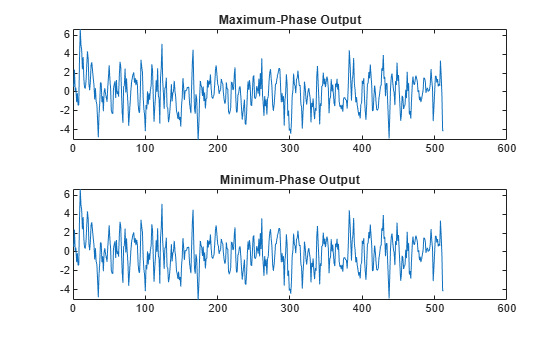# latcfilt

## Syntax

``` [f,g] = latcfilt(k,x) [f,g] = latcfilt(k,v,x) [f,g] = latcfilt(k,1,x) [f,g,zf] = latcfilt(...,'ic',zi) [f,g,zf] = latcfilt(...,dim) ```

## Description

` ` When filtering data, lattice coefficients can be used to represent

• FIR filters

• All-pole IIR filters

• Allpass IIR filters

• General IIR filters

`[f,g] = latcfilt(k,x)` filters `x` with the FIR lattice coefficients in the vector `k`. The forward lattice filter result is `f` and `g` is the backward filter result. If $|k|\le 1$, `f` corresponds to the minimum-phase output, and `g` corresponds to the maximum-phase output.

If `k` and `x` are vectors, the result is a (signal) vector. Matrix arguments are permitted under the following rules:

• If `x` is a matrix and `k` is a vector, each column of `x` is processed through the lattice filter specified by `k`.

• If `x` is a vector and `k` is a matrix, each column of `k` is used to filter `x`, and a signal matrix is returned.

• If `x` and `k` are both matrices with the same number of columns, then the ith column of `k` is used to filter the ith column of `x`. A signal matrix is returned.

`[f,g] = latcfilt(k,v,x)` filters `x` with the IIR lattice coefficients `k` and ladder coefficients `v`. Both `k` and `v` must be vectors, while `x` can be a signal matrix.

`[f,g] = latcfilt(k,1,x)` filters `x` with the IIR lattice specified by `k`, where `k` and `x` can be vectors or matrices. `f` is the all-pole lattice filter result and `g` is the allpass filter result.

`[f,g,zf] = latcfilt(...,'ic',zi)` accepts a length-`k` vector `zi` specifying the initial condition of the lattice states. Output `zf` is a length-`k` vector specifying the final condition of the lattice states.

`[f,g,zf] = latcfilt(...,dim)` filters `x` along the dimension `dim`. To specify a `dim` value, the FIR lattice coefficients `k` must be a vector and you must specify all previous input parameters in order. Use the empty vector [ ] for any parameters you do not want to specify. `zf` returns the final conditions in columns, regardless of the shape of `x`.

## Examples

collapse all

Generate a signal with 512 samples of white Gaussian noise.

`x = randn(512,1);`

Filter the data with an FIR lattice filter. Specify the reflection coefficients so that the lattice filter is equivalent to a 3rd-order moving average filter.

`[f,g] = latcfilt([1/2 1],x);`

Plot the maximum- and minimum-phase outputs of the lattice filter in separate plots

```subplot(2,1,1) plot(f) title('Maximum-Phase Output') subplot(2,1,2) plot(g) title('Minimum-Phase Output')```